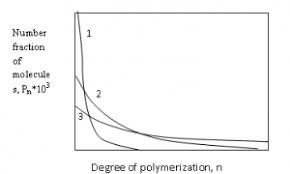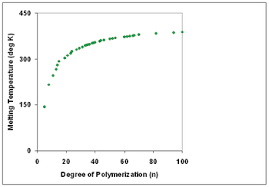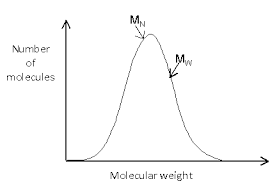## How to Calculate and Solve for Degree of Polymerization | PolymerThe image of degree of polymerization is represented above.

To compute for degree of polymerization, two essential parameters are needed and these parameters are Mean Number Molecular Weight (M’nand Unit Molecular Weight (m).

The formula for calculating degree of polymerization:

D.P. = M’n/m

Where:

D.P. = Degree of Polymerization
M’n = Mean Number Molecular Weight
m = Unit Molecular Weight

Let’s solve an example;
Find the degree of polymerization when the mean number molecular weight is 28 and the unit molecular weight is 4.

This implies that;

M’n = Mean Number Molecular Weight = 28
m = Unit Molecular Weight = 4

D.P. = 28/4
D.P. = 7

Therefore, the degree of polymerization is 7.

Calculating the Mean Number Molecular Weight when the Degree of Polymerization and the Unit Molecular Weight is Given.

M’n = D.P (m)

Where;

M’n = Mean Number Molecular Weight
D.P. = Degree of Polymerization
m = Unit Molecular Weight

Let’s solve an example;
Find the mean number molecular weight when the degree of polymerization is 6 and the unit molecular weight is 4.

This implies that;

D.P. = Degree of Polymerization = 6
m = Unit Molecular Weight = 4

M’n = D.P (m)
M’n = 6 (4)
M’n = 24

Therefore, the degree of polymerization is 24.

## How to Calculate and Solve for Degree of Polymerization | combination | Polymer & TextileThe image above represents the degree of polymerization.

To compute for degree of polymerization, one essential parameter is needed and this parameter is parameter (V).

The formula for calculating the degree of polymerization:

Xn = 2V

Where:

Xn = Degree of Polymerization
V = Parameter

Let’s solve an example;
Find the degree of polymerization with a parameter of 28.

This implies that;

V = Parameter = 28

Xn = 2V
Xn = 2 (28)
Xn = 56

Therefore, the degree of polymerization is 56.

## How to Calculate and Solve for Molecular Weight of Homopolymer | Polymer & TextileThe image above represents the molecular weight of homopolymer.

To compute for molecular weight of homopolymer, two essential parameters are needed and these parameters are Degree of Polymerization (x) and Sum of Masses of Repeat Unit (Mo).

The formula for calculating the molecular weight of homopolymer:

M = xMo

Where;

M = Molecular Weight of Homopolymer
x = Degree of Polymerization
Mo = Sum of Masses of Repeat Unit

Let’s solve an example;
Given that the degree of polymerization is 12 and the sum of masses of repeat unit is 24. Find the molecular weight of homopolymer?

This implies that;

x = Degree of Polymerization = 12
Mo = Sum of Masses of Repeat Unit = 24

M = xMo
M = (12)(24)
M = 288

Therefore, the molecular weight of the homopolymer is 288.

Calculating the Degree of Polymerization when the Molecular Weight of Homopolymer and the Sum of Masses of Repeat Unit is Given.

x = M / Mo

Where;

x = Degree of Polymerization
M = Molecular Weight of Homopolymer
Mo = Sum of Masses of Repeat Unit

Let’s solve an example;
Given that the molecular weight of homopolymer is 140 and the sum of masses of repeat unit is 70. Find the degree of polymerization?

This implies that;

M = Molecular Weight of Homopolymer = 140
Mo = Sum of Masses of Repeat Unit = 70

x = M / Mo
x = 140 / 70
x = 2

Therefore, the degree of polymerization is 2.